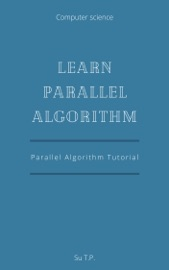# Books: Learn Parallel Algorithm

Learn Parallel Algorithm
Parallel Algorithm Tutorial

A parallel algorithm can be executed simultaneously on many different processing devices and then combined together to get the correct result. Parallel algorithms are highly useful in processing huge volumes of data in quick time. This tutorial provides an introduction to the design and analysis of parallel algorithms. In addition, it explains the models followed in parallel algorithms, their structures, and implementation.
This tutorial will help the undergraduate students of computer science learn the basic-to-advanced topics of parallel algorithm.
In this tutorial, all the topics have been explained from elementary level. Therefore, a beginner can understand this tutorial very easily. However if you have a prior knowledge of writing sequential algorithms, it will be helpful in some chapters.Title : Learn Parallel Algorithm.pdf Author : Su T.P Size : 903.25 KiB Category : Computers, Books, Computers & Internet Format Type : eBook PDF / e-Pub Date release : 2020-07-07